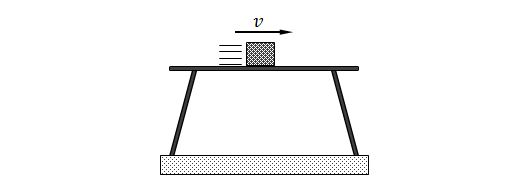Classical Mechanics

# Identifying Forces

The Mission Impossible movies are typically filled with stunts that live on the edge of what seems possible. In the recent movie Ghost Protocol, one stunt involves an agent wearing a funky high-tech metal suit (it looks like some sort of chainmail). The agent is levitated off the floor using magnetic forces between the agent and a small robot sitting on the ground.

If I wanted to suspend a $90~\mbox{kg}$ secret agent in midair, what vertical force in Newtons must I exert on the agent, electromagnetic or otherwise?

Details and assumptions

• The acceleration due to gravity is $-9.8~\mbox{m/s}^2$.A steel ball hangs from the ceiling by a spring. If the mass of the steel ball is $8\text{ kg}$ and that of the spring is $500\text{ g},$ which of the following forces is acting on the steel ball when it is at rest (at its equilibrium position)?

A middle school student Sam just learned gravity from Science class. He looks at a plate on a table and thinks, 'The plate is being pulled by the Earth. Therefore, it should fall to the Earth now. But why does it stay on the table?'

What is the answer to Sam's question?

A steel cannon ball is moving in parabolic motion after it was fired from a cannon. Assume that the cannon ball is in a vacuum. What is the name of the only force acting on the cannon ball?A block slides across the top of a smooth table with a velocity of $v.$ Identify the force on the block.

×